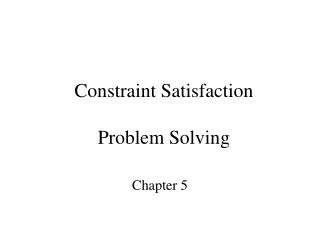# Constraint Satisfaction Problem Solving - PowerPoint PPT PresentationDownload PresentationConstraint Satisfaction Problem Solving

Constraint Satisfaction Problem SolvingDownload Presentation## Constraint Satisfaction Problem Solving

- - - - - - - - - - - - - - - - - - - - - - - - - - - E N D - - - - - - - - - - - - - - - - - - - - - - - - - - -
##### Presentation Transcript

1. Example problems Illustrative • Map coloring • Cryptarithmetic • N-queens Real • Class scheduling • Scheduled Hubble telescope • Discrete Optimization problems

2. Goal: solve the problem Find bindings for all the variables that satisfy all the constraints. Corresponds to boolean satisfiability, except that now variables take on values from discrete sets.

3. Formal Model • Finite set of variables: X1,…Xn • Variable Xi has values in finite domain Di. • Constraints C1…Cm. A constraint specifies legal combinations of the values. Tables. • Assignment: selection of values for all variables. • Consistent: assignment satisfies all constraints.

4. Note: Constraints can be made binary • Constraints = table of legal values • One trinary table between(a,b,c). • Between(2,4,5) • Replaced by conjunction of two binary relations • Less(2,4) & Less(4,5)

5. C1 C2 C3 Simple Mapcolors: red, blue, greenGoal: assign colors so touching countries have different colors

6. Model • Variables X1, X2, X3 • Domains Di = {red,blue,green}. • X1 !=X2 Table with 6 entries:

7. Naïve BackTracking Solution • X1 = red, X2= red, X3 = red, X1!=X2 #Fail • Short hand for table lookup (not in table) • X1= red, X2=red, X3=blue, X1!=X2 #Fail • X1= red, X2=red, X3=green….Fail • X1= red, X2=blue,…. Continue to Solution.. UGH. But it is the way Prolog works.

8. Selections in Backtracking • Selecting a variable to bind • Selecting a value to assign to the variable Does Order Matter? • Yes and no • No: search is complete: any ordering will find a solution if one exists. • Yes: search cost changes.

9. Controlling Backtracking • Choosing a variable to bind • Minimum remaining values (MRV) • Intuition: need to solve eventually so do hardest case first • Degree Heuristic • choose variable involved in largest number of constraints. (tie-breaker) • Choosing a binding/value for variable • Least constraining variable • Intuition: maximize search options

10. Forward Checking • Constraint Propagation • If variable X is bound, find all variables Y that are connected to it by a constraint. Eliminate values that are inconsistent. • Can yield dramatic improvement. • Provably better than backtracking • Guaranteed to do less work

11. Relook at Map-coloringUsing Forward Checking X1= red: remove red from X2 and X3 domains X2 = blue: remove blue from X3’s domain X3 = green. Done!

12. Arc-Consistency (3) • Binary CSP (can always force this) • Arc = edge between variables that are in same constraint. • Let X-Y be arc. • for each x in Domain X if there is no value y in domain Y that allows constraint to be met, then delete x from domain X. • Just look in the tables • Can be done as preprocessing or during search.

13. AC3 Example • TWO+TWO = FOUR • edge between T and F gives: • (T+T+Carry )/10 = F we see that only T in {5,6,7,8,9} can work for F = 1. • Edge between F and R says R \= 1. • Edge between (O+O)%10 = R and O\=R gives: O \= 0.